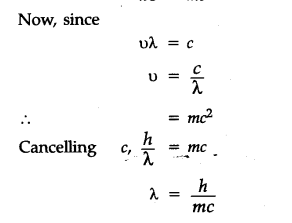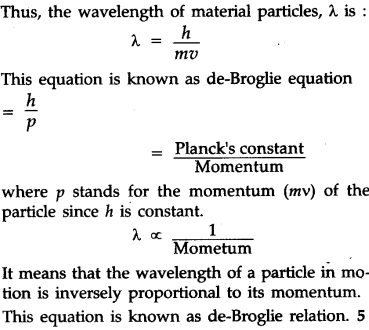# Derive the derivation of de-Broglie relationship

The relationship may be derived by combining the mass-energy relationships proposed by Max Plane and Einstein.
According to Planck, photon of light having energy E is associated with a wave of frequency o as:
E=h\$\upsilon According to Einstein, mass and energy are related as : E=m{{c}^{2}} where c is the velocity of light Combining the above two relations in equation (i) and (ii), we get : h\upsilon =m{{c}^{2}}\$The equation is valid for a photon. de-Broglie suggested that on substituting the mass of the particle m and its velocity v in place of velocity of light c, the equation can also be applied to material particles.